# Sag and Tension in overhead transmission line

SAG AND TENSION

Mechanical structures consisting of components such as insulator, cross arms, poles or tower supports the overhead line. The structures must be mechanically strong​​ even under the worst weather conditions. The conductor is acted by the forces such as weight of the conductor itself, wind pressure and tension. The tension in the conductor is normally expected to be less than 50% of its ultimate tensile strength even when there is 12.7mm radial coating of ice and a wind pressure of the order of 380 N/m^2.The tension in the conductor depends on the diameter of the conductor, length of the conductor between supports, material of conductor, sag in conductor, wind pressure and temperature. Sag and tension depends upon the loading conditions and temperature variations. When the temperature decreases tension increases and sag decreases.

SAG

The difference in level between the points of supports and the lowest point on the conductor is known as sag. If the sag is very small compared to the span, its shape is parabola. The factor affecting the sag in the overhead transmission line are as follows:

• Weight of the conductor: Heavier the conductor, greater will be the sag. In case of ice formation takes place in line cause increase in sag.

• Length of the span: sag is directly proportional to the square of the span length .in case of working tension, temperature with longer span will have greater sag.

• Working Tensile strength: Sag is inversely proportional to the working tensile strength of the conductor if and only temperature, length of span remains same.

• Temperature:​​ When the metallic bodies expand with the rise in temperature the length of the conductor increases and with rise in temperature sag increases.

Sag is the important factor for the design of overhead line. In case of more sag we need more conductor material, more weight on the supports is to be supported, higher supports are necessary and there is a chance of greater swing-amplitude due to wind load.in case of less sag, there is more tension in the conductor and conductor break if any additional stress is to be taken.so mainly two condition should be keep into account for sag-tension calculations:

• At minimum Temperature: The lowest sag and maximum tension in conductor occurs when the temperature is minimum and wind maximum.

• At maximum Temperature: Maximum sag occurs when the temperature is maximum and there is no wind pressure.​​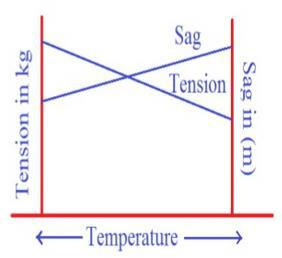Condition Tension Sag Weight of​​ conductor per unit Temperature Ice Wind Toughest condition(T1) Maximum Minimum W1 Minimum(0°c) Yes Yes Stringing condition​​ Normal condition(T2) Normal Normal W2=Wc 25°c No No Easiest condition(T3) Minimum Maximum W3=Wc Maximum(65°c) NO No

𝑇1 =​​ 𝑇𝑜𝑢𝑔𝑒𝑠𝑡​​ 𝑡𝑒𝑛𝑠𝑖𝑜𝑛​​ 𝑐𝑜𝑛𝑑𝑖𝑡𝑖𝑜𝑛

​​  Minimum temperature​​ 𝜃1​​

 Maximum wind

​​

𝑇2​​ =​​ 𝑇𝑠𝑡𝑟𝑖𝑛𝑔𝑖𝑛𝑔​​

i. Ambient temperature​​ 𝜃2​​

ii. No wind

​​ iii. No ice​​

​​

𝑇3 =​​ 𝐸𝑎𝑠𝑖𝑒𝑠𝑡​​ 𝑠𝑎𝑔𝑔𝑖𝑛𝑔​​ 𝑐𝑜𝑛𝑑𝑖𝑡𝑖𝑜𝑛

​​  Maximum allowable temperature

​​  No wind

​​  No Ice

Tensioning, Stringing and Sagging:​​

 Tension in conductor during operation varies for a given conductor at a given span.

​​ ​​ 𝑇𝑚𝑎𝑥​​ ≤ UTS / FS is limiting factor ​​

​​ 𝑇𝑚𝑖𝑛​​ = Sag​​ is the limiting factor​​

​​

Calculation of sag

S=WL^2/T

Where w=weight of conductor in kg meter length,

L=length of the span in meters, and

T=tension in the conductor in Kg.

Calculation of sag and Tension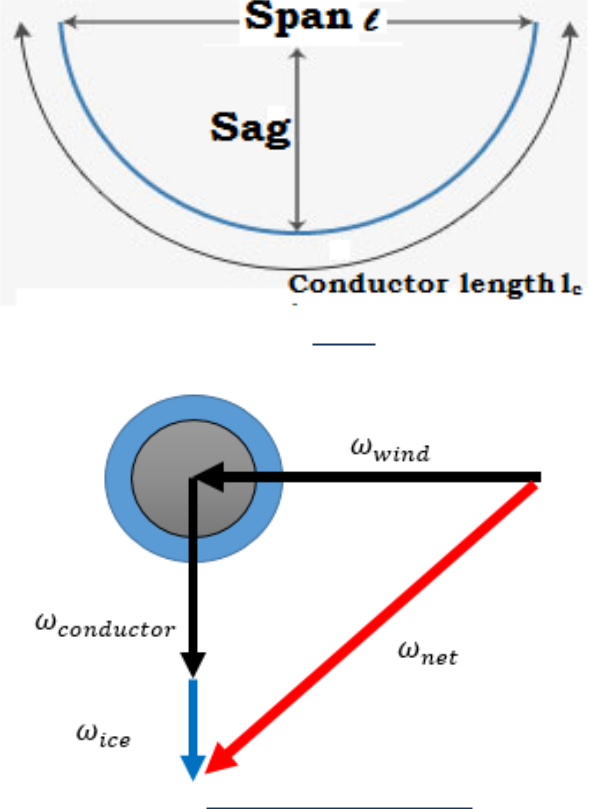Supports at same level​​

Consider​​ a transmission line with equal span length of L ,the total conductor length between supports be l, the horizontal tension at lowest point o be H ,the conductor weight per unit length w and the tension along the conductor curve be T at any point p. Also consider the curve distances s from o to p such that x be the horizontal distance and y be vertical distance. The tension at p can be resolved and found,​​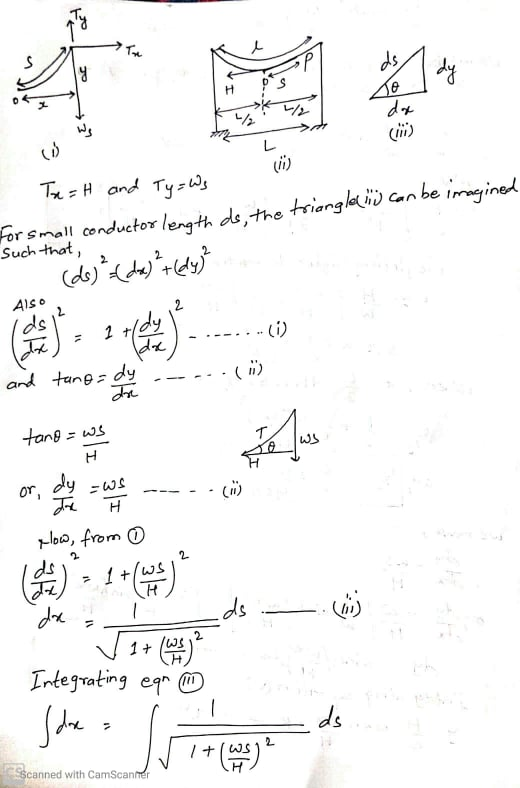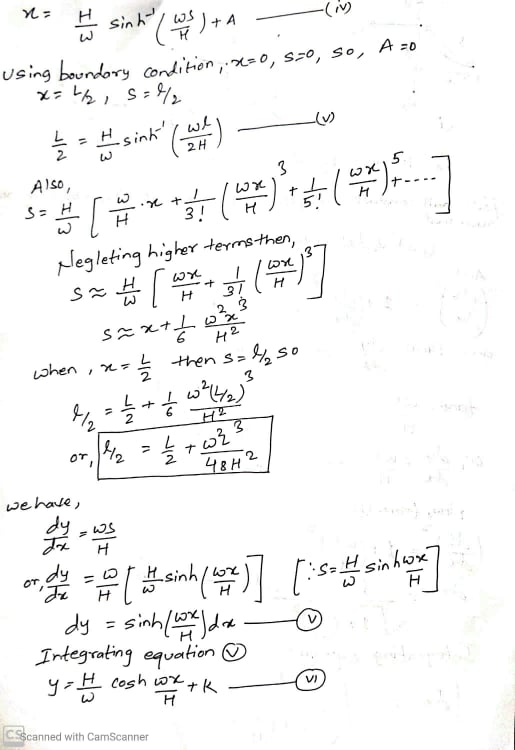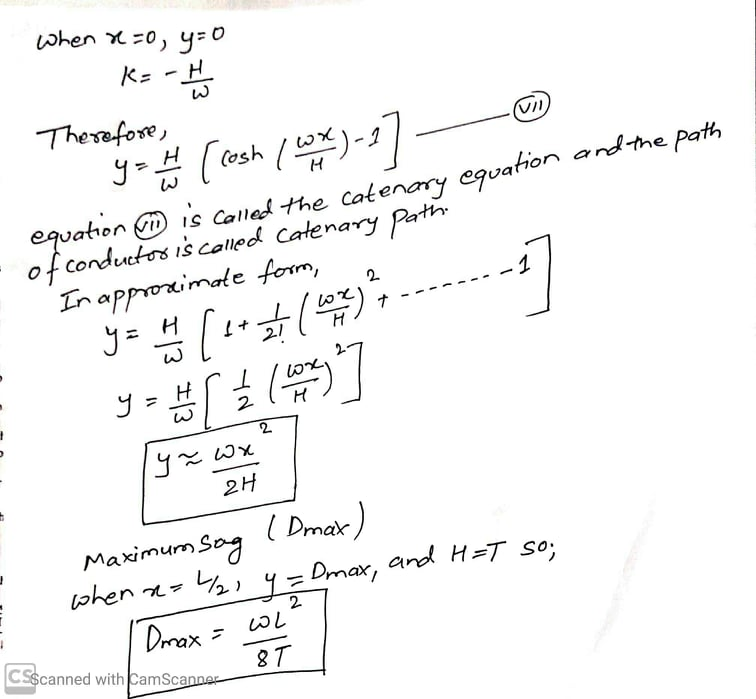Supports at unequal level

AOB = conductor that has point lowest point O

L = Span of the conductor.

h = difference in height level between two supports

T = tension of the conductor.

w = weight per unit length of the conductor.

𝑥1 = distance of support at lower level point A form O.​​

𝑥2 = distance of support at upper level point B from O.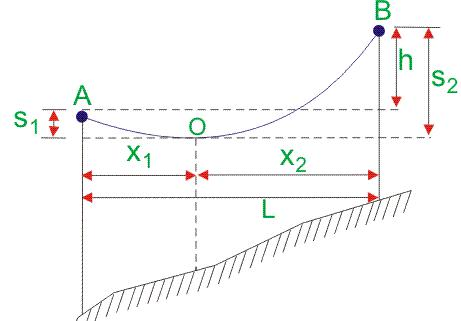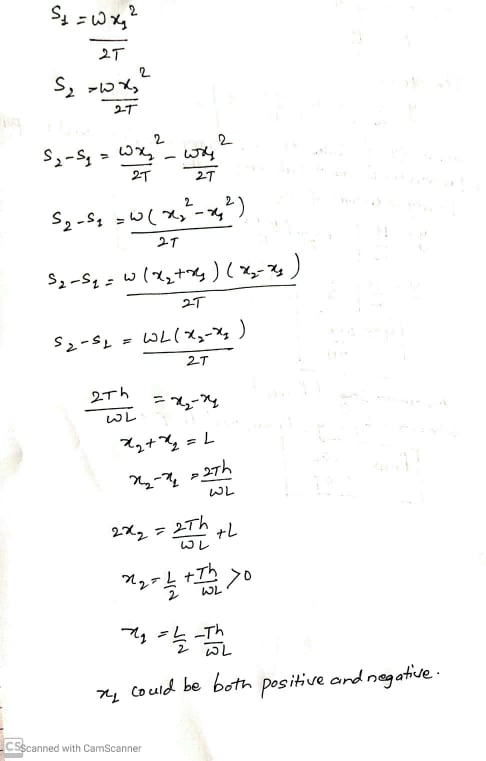Virtual sag

In above, when h>>L, such that L/2<(Th/wL) so the value of x1 is found to be negative i.e x1<0.This means that the lowest point of conductor lies outside of the span and is a virtual condition ,this is called virtual sag.

Rulling Span

When​​ the span length for each section ae different than the equivalent span length is used and is called ruling span. mathematically rulling span is,

Le=((L1^3+L2^3+…………+Ln^n)/(L1+L2+………..+Ln))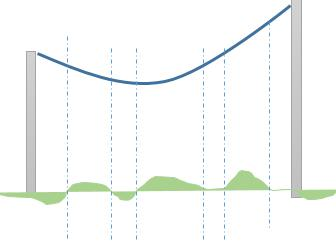Effect of wind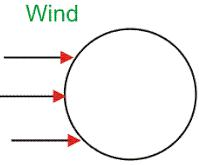Wind force on conductor:​​ 𝑭𝒘𝒇𝒄𝒓 ​​​​

𝑭𝒘𝒇𝒄𝒓= Projected area of conductor × wind pressure (kg/𝑚3) ​​

Per conductor projected area of circular surface in geometry = 2 /3 *dcr ×span length ​​

​​ dcr = Diameter of conductor​​

​​ For single conductor:​​

​​

𝐵𝑒𝑛𝑑𝑖𝑛𝑔​​ 𝑀𝑜𝑚𝑒𝑛𝑡​​ 𝐵𝑀​​ =​​ 𝑭𝒘𝒇𝒄𝒓​​ ×(1 +2 +3)

For​​ 𝑁𝑐​​ Circuit:​​

​​

𝑩𝒆𝒏𝒅𝒊𝒏𝒈​​ 𝑴𝒐𝒎𝒆𝒏𝒕​​ 𝑩𝑴​​ =​​ 𝑭𝒘𝒇𝒄𝒓​​ ×𝑵𝒄​​ ×(𝒉𝟏​​ +𝒉𝟐​​ +𝒉𝟑) + ​​ 𝟐𝑻​​ 𝐬𝐢𝐧​​ 𝜶/​​ 𝟐​​ ×𝑵𝒄​​ ×(𝒉𝟏​​ +𝒉𝟐​​ +𝒉𝟑) +​​ 𝑭𝒘𝒇𝒄𝒓​​ ×𝑵𝑬𝒘​​ ×𝑯​​ +​​ 𝟐𝑻𝑬𝑾​​ 𝐬𝐢𝐧​​ 𝜶/​​ 𝟐​​ ×𝑵𝑬𝒘​​ ×𝑯​​

​​ T = worst condition​​ tension so take​​ 𝑇𝑚𝑎𝑥​​ =​​ 𝑈𝑇𝑆/​​ 𝐹𝑆

UTS=ultimate tensile strength (For a given particular condition)

FS=Factor of safety (2-2.5)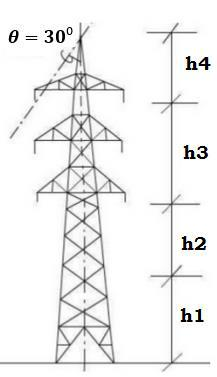h1=minimum permissible ground clearance

h2=maximum sag

h3=vertical spacing between conductors

h4=vertical​​ clearance between earth wire and top conductor

Effect of ice​​

There is the possibility of formation of ice coating in the line conductor where the temperature drops below. There is increase in weight and effective diameter of the conductor due to ice coating in the line conductor. The weight of ice together with the weight of line conductor acts downwards. Thus the total weight acting vertically on the conductor per meter length is Wc+Wi; where Wc is the weight of conductor in kg per meter length and Wi is the weight of ice per meter length.

Wi can be determined as follows: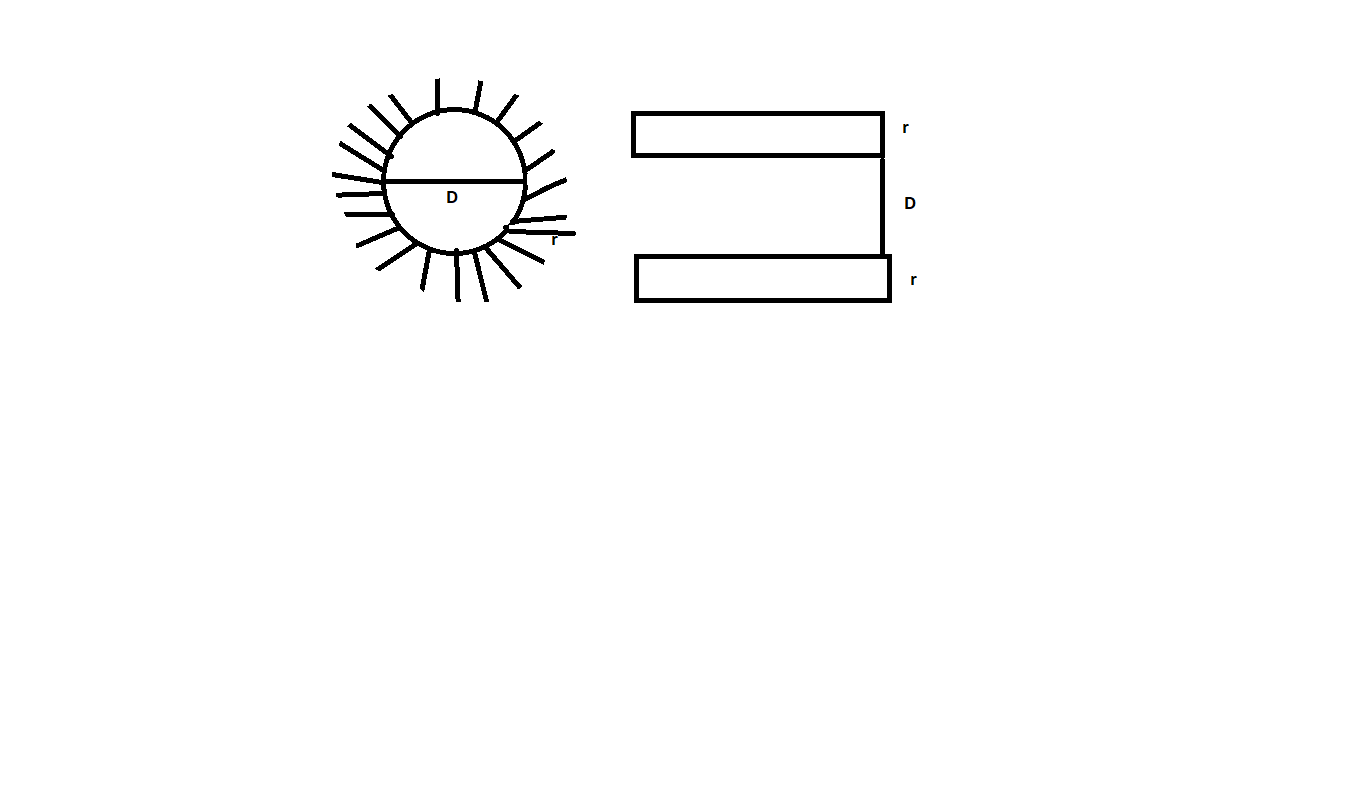Let D be the diameter of conductor in meters and radial thickness of ice coating be r meters. The overall diameter is (D+2r).

Volume of ice coating per meter length of conductor​​

=π/4*[(D+2r) ^2-D^2]

= π/4*[4Dr+4*r^2]

= πr(D+r) m^3

The density of ice is approximately 920Kg per m^3,

The weight of ice coating per meter length,

Wi=920* πr(D+r) Kg

=2890.3r(D+r) kg/m

Combine effects of wind and ice

Due to weight of ice deposits on the line,​​ and the wind pressure, the mechanical stress increases in the conductor and therefore the line must be designed to withstand stresses and tensions. The total weight of ice together with the weight of conductor acts vertically downwards while the wind loading Ww acts horizontally,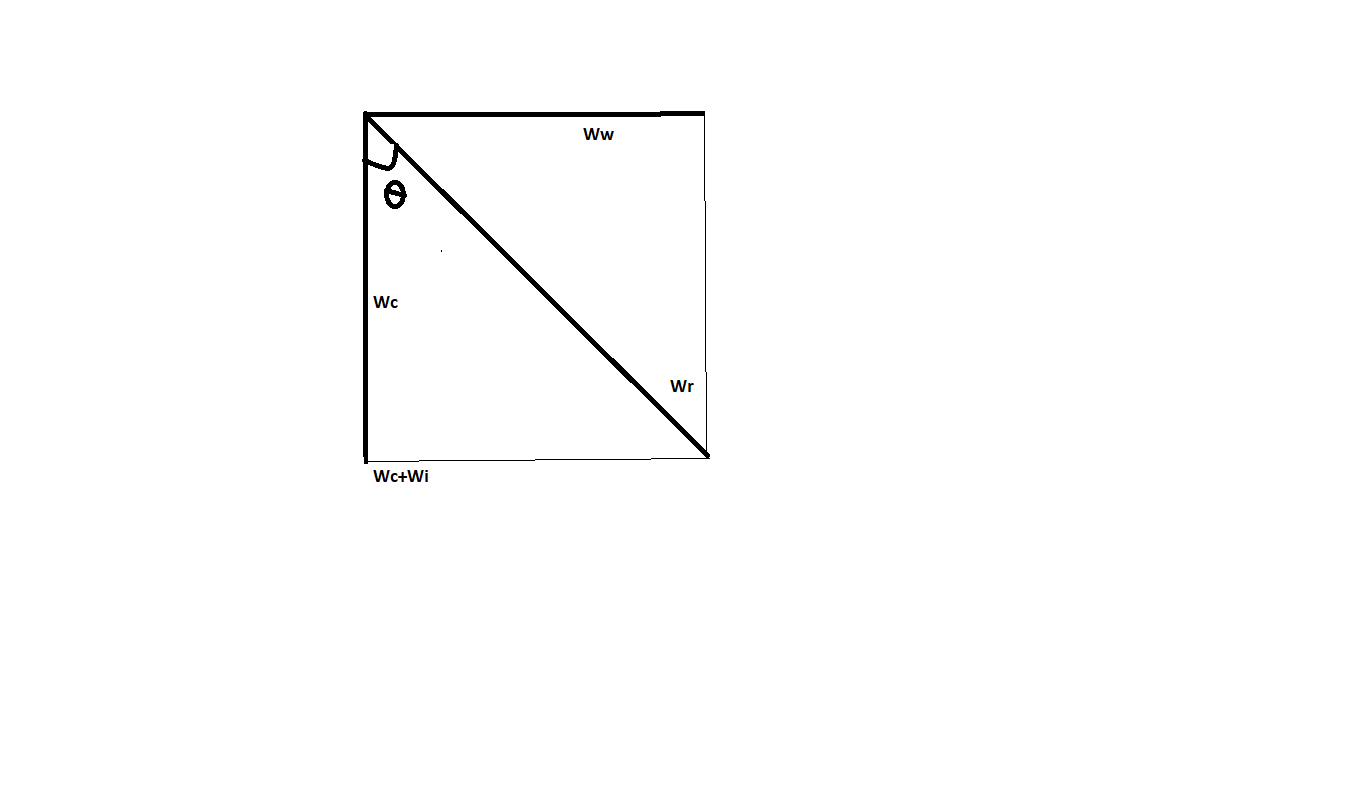Resultant weight per meter length of conductor =(Wc+Wi2+Ww2)

Where,

Ww=wind force in Kg per meter length

=wind pressure per m^2 of projected area*projected area per meter length

=P*(D+2r)

Maximum sag=(WrL^2)/8T……….(slant slag)

When the​​ ice and wind are acting ,the lowest point of the conductor does not remain vertically down but away from it at an angle​​ ϴ.

The vertical sag can be obtained by multiplying the slant sag with cos​​ ϴ.

### 1 thought on “Sag and Tension in overhead transmission line”

1.